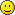RandonInt()RandonInt()

A nested function call is when inside a function, you call the same function.\

We will now define a function RandomInt(N). This function generates a random number between 0 and N-1 (inclusive) with equal probability.
Now RandomInt(Random(Random(..........RandomInt(N).....)))) is nested.
RandomInt(N) means nesting of 1.
And RandomInt(RandomInt(N)) means nesting of 2 and thus so on....

You will be given a Number N, nesting (the no of nestings) and Target.

You have to give the probability of getting a number = Target after nestings starting from number N

SwitchCase

Posts : 5
Join date : 2008-08-13Re: RandonInt()

I'm not able to figure out probability but considered following cases:
Let N be number, T be target and n be nestings
if n>=N and T>0 we cannot get T
if N>T (alsothen we cannot get T
if n> N-T ( N > T obviously ) ,we cannot get T
else
we can get T with probability is as follow
0: 1/N +1/2N + ....+ 1/nN
1:1/N +.........+ 1/(n-1)N
.
.
N-1: 1/Nankurgutpa

Posts : 44
Join date : 2008-08-10
Age : 32
Location : Tandon 72Re: RandonInt()

my understanding of the problem is simple(probably not right).

target > N - number_of_nestings OR target < 0
P(target) = 0

0<= target <= N - number_of_nestings
this part needs more maths.... i am weak in it..
its not evenly distributed of course.because at the end,the outer most RandInt can get anything less than N - number_of_nestings as input.
so intuitively it feels like P(1) > P(2) > P(3) .....
somebody please solve this thing!!!

Last edited by Ramprasadg on Tue Oct 14, 2008 9:09 pm; edited 1 time in totalPosts : 12
Join date : 2008-08-13Re: RandonInt()

P(N-1) != 1/N

see to get N-1 as target,every nested RandomINt must give N-1.
so the ans is (1/N-1)^no_of_nestings.Posts : 12
Join date : 2008-08-13Re: RandonInt()